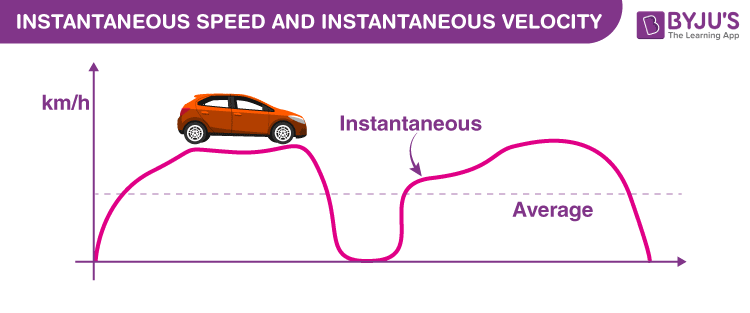# Instantaneous Speed and Instantaneous Velocity

Instantaneous velocity is defined as the rate of change of position for a time interval which is very small (almost zero). Measured using SI unit m/s. Instantaneous speed is the magnitude of the instantaneous velocity. It has the same value as that of instantaneous velocity but does not have any direction.

## Instantaneous Velocity

In simple words, the velocity of an object at that instant of time. Instantaneous velocity definition is given as “The velocity of an object under motion at a specific point of time.”

If the object possesses uniform velocity, then the instantaneous velocity may be the same as its standard velocity.It is determined very similarly as that of average velocity, but here the time period is narrowed. We know that the average velocity for a given time interval is total displacement divided by total time. As this time interval approaches zero, the displacement also approaches zero. But the limit of the ratio of displacement to time is non-zero and is called instantaneous velocity.

## Instantaneous Velocity Formula

The instantaneous velocity formula is given by

 $$\begin{array}{l}V_{i}=\lim_{\Delta t\rightarrow 0}\frac{ds}{dt}\end{array}$$

Where,

• Δt is the small-time interval.
• Vi is the instantaneous velocity.
• s is the displacement.
• t is the time.

### Unit of Instantaneous Velocity

The SI unit of instantaneous velocity is m/s. It is a vector quantity. It can also be determined by taking the slope of the distance-time graph or x-t graph.

## Instantaneous Velocity Example

If the displacement of the particle varies with respect to time and is given as (6t2 + 2t + 4) m, the instantaneous velocity can be found out at any given time by:

s = (6t2 + 2t + 4)

Velocity (v) =

$$\begin{array}{l} \frac {ds}{dt} \end{array}$$
$$\begin{array}{l} \frac{d(6t^2 + 2t + 4)}{dt}\end{array}$$
= 12t + 2

So, if we have to find out the instantaneous velocity at t = 5 sec, then we will put the value of t in the obtained expression of velocity.

Instantaneous velocity at t = 5 sec = (12×5 + 2) = 62 m/s

Let us calculate the average velocity now for 5 seconds now.

Displacement = (6×52 + 2×5 + 4) = 164 m

Average velocity =

$$\begin{array}{l} \frac{164}{5}\end{array}$$
= 32.8 m/s

## Instantaneous Speed

We know that the average speed for a given time interval is the total distance travelled divided by the total time taken. As this time interval approaches zero, the distance travelled also approaches zero. But the limit of the ratio of distance and time is non-zero and is called the instantaneous speed. To understand it in simple words, we can also say that instantaneous speed at any given time is the magnitude of instantaneous velocity at that time.

## Instantaneous Speed Formula

The instantaneous speed formula is given by

 $$\begin{array}{l}Speed_{(i)}=\frac{ds}{dt}\end{array}$$

Where,

• ds is the distance
• dt is the time interval
• Speed(i) is the instantaneous speed

### Unit of Instantaneous Speed

The SI unit of instantaneous speed m/s. It is a scalar quantity. Instantaneous velocity can be linear velocity or angular velocity.

## Instantaneous Speed Example

If distance as a function of time is known to us, we can find out the instantaneous speed at any time. Let’s understand this by the means of an example.

Let Distance (s) = 5t3 m

Substitute it in the formula

$$\begin{array}{l}Speed_{(i)}=\frac{ds}{dt}\end{array}$$
we get-

$$\begin{array}{l}Speed_{(i)}=\frac{d5t^{3}}{dt}\end{array}$$

= 15t²
We can now easily find the instantaneous speed at any given time by putting the value of t in this obtained expression.

### Difference between Instantaneous Speed and Instantaneous Velocity

 Instantaneous speed Instantaneous velocity Instantaneous speed is the magnitude of instantaneous velocity Instantaneous velocity is the change in position taking place at a small change in time Instantaneous speed is a scalar quantity Instantaneous velocity is a vector quantity Formula of instantaneous speed is $$\begin{array}{l}Speed_{(i)}=\frac{ds}{dt}\end{array}$$ Formula of instantaneous velocity is $$\begin{array}{l}V_{i}=\lim_{\Delta t\rightarrow 0}\frac{ds}{dt}\end{array}$$

Following is the table of links related to the difference between concepts:

## Practice Questions on Instantaneous Speed And Instantaneous Velocity

Q1: Define instantaneous velocity.

Ans: The velocity of an object under motion at a specific point of time.

Q2: Write the units of instantaneous velocity.

Ans: The units of instantaneous velocity is m/s.

Q3: Is instantaneous velocity a scalar or vector?

Ans: Instantaneous velocity is a vector quantity.

Q4: Write the expression for instantaneous velocity.

Ans: The formula of instantaneous velocity is given by-

$$\begin{array}{l}V_{i}=\lim_{\Delta t\rightarrow 0}\frac{ds}{dt}\end{array}$$

Q5: If the object moves with a constant standard velocity of 50 km/hr for two hours. What is its instantaneous velocity after 30 minutes from the time it started?

Ans: Instantaneous velocity of the object is 50 km/hr. As the object is under uniform velocity, its instantaneous velocity is the same as its standard velocity.

Q6: Define instantaneous speed.

Ans: Speed of the object at a particular moment or instant of time.

Q7: Write the expression for instantaneous speed.

Ans: The instantaneous speed formula is given by

$$\begin{array}{l}Speed_{(i)}=\frac{ds}{dt}\end{array}$$
.

Q8: If the object has the instantaneous velocity of 40 miles per hour. What is its instantaneous speed?

Ans: The instantaneous speed of the object is 40 miles per hour.

Q9: How are instantaneous velocity and instantaneous speed related?

Ans: Instantaneous Speed is the magnitude of the Instantaneous velocity.